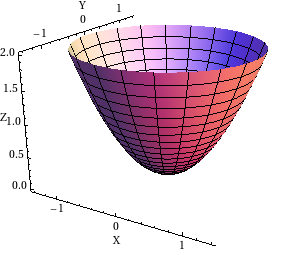# Math Insight

### Image: Elliptic paraboloid on a circular domainGraph of the elliptic paraboloid $z=x^2+y^2$ plotted on the circular domain $x^2+y^2 \le 2$.

Image file: elliptic_paraboloid_circular_domain.png

Source image file: elliptic_paraboloid_circular_domain.nb
Source image type: Mathematica